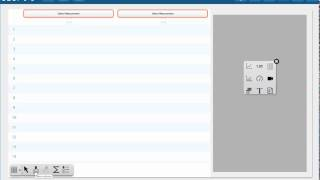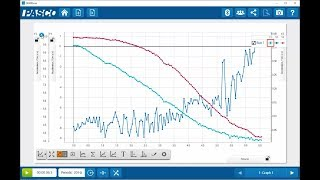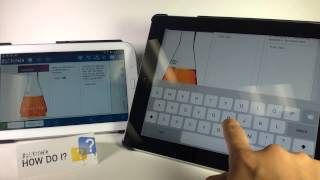# Plot a Circle (SPARKvue)

How do I draw a circle within SPARKvue? Because a circle is not a function, we need to use a parametric equation to draw the circle. Using the calculator, we can draw parametric equations within SPARKvue, and then use the tools within SPARKvue to explore the properties of the figure.

### More "How Do I?" Videos